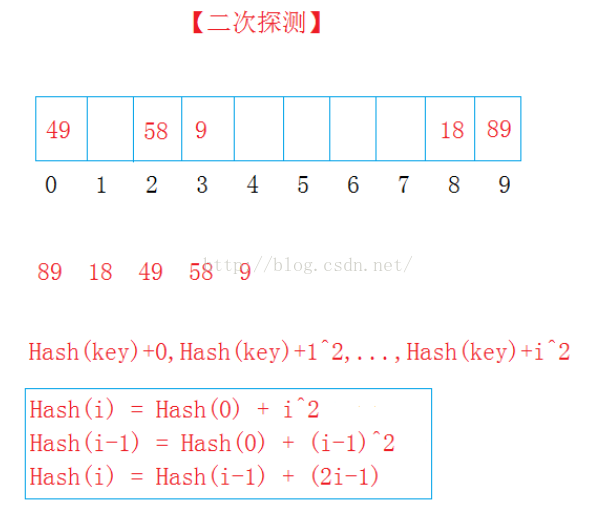# 第七章学习小结

（1）左子树的所有结点均小于根的值；
（2）右子树的所有结点均大于根的值；
（3）它的左右子树也分别为二叉排序树。Hash（key） = key % 10（表长）；
89放入9的位置；18放入8的位置；49与89冲突，加1^2，到0的位置；58与18冲突，加1^2,到了9的位置，有数继续加2^2到2的位置；9与89冲突，加1^2，在0的位置，继续加2^2，到3的位置放入。
Hash（key）+ i^2（i= 1、2、3……不算第一放入的数）

7-1 Hashing (30 分)

The task of this problem is simple: insert a sequence of distinct positive integers into a hash table, and output the positions of the input numbers. The hash function is defined to be ( where

Note that the table size is better to be prime. If the maximum size given by the user is not prime, you must re-define the table size to be the smallest prime number which is larger than the size given by the user.

### Input Specification:

Each input file contains one test case. For each case, the first line contains two positive numbers:

### Output Specification:

For each test case, print the corresponding positions (index starts from 0) of the input numbers in one line. All the numbers in a line are separated by a space, and there must be no extra space at the end of the line. In case it is impossible to insert the number, print "-" instead.

### Sample Input:

4 4
10 6 4 15


### Sample Output:

0 1 4 -#include <iostream>
#include <math.h>

using namespace std;

int prime(int m)//验证一个数是不是素数
{
if(m == 1)
return 0;
int i = 2, n;
n = sqrt(m)+1; //n的平方数+1
while(i < n)
{
if(m%i==0)  //整除，直接返回函数值0
return 0;
i++;
}
if(i==n) //非整除退出循环，i肯定等于n
return 1;
}

int GetNextPrime(int x)
{ //先判断是否为素数，不是则+1，以此循环
int i = x;
while(1)
{
if(prime(i))  //若是素数，退出循环
break;
i++;
}

return i;
}

int Hash(int key, int TableSize)
{   // 获取映射
return key % TableSize;
}

int main()
{
int TableSize, N, pos, tempos;
cin >> TableSize >> N;

TableSize = GetNextPrime(TableSize);

int A[TableSize], Data[N];

for(int i=0; i<TableSize; i++)//先将A数组内所有值赋为0
A[i] = 0;

for(int i=0; i<N; i++)//输入数值
cin >> Data[i];

for(int i=0; i<N; i++)
{
if(i != 0)//控制好空格的输出
cout << " ";
pos = Hash(Data[i], TableSize);
tempos = pos;//先将pos值付给tempos
if(A[pos] == 0)
{//如果未被访问
A[pos] = Data[i];
cout << pos;
}
else
{//如果已被访问
int j, flag=0;
for(j=1; j<TableSize; j++)
{
pos = Hash(tempos+j*j, TableSize);
if(A[pos] == 0)
{
flag = 1;//做好标志
A[pos] = Data[i];
cout << pos;
break;
}
}
if(flag == 0)
cout << "-";
}
}
}
View Code

posted @ 2019-06-02 22:23  07-14  阅读(179)  评论(0编辑  收藏  举报